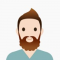## Program to find smallest difference of angles of two parts of a given circle in C++Ayush Gupta

Updated on 16-May-2022 07:31:19

In this problem, we are given an array that denotes the piece of a circle based on the angles of a circle. Our task is to create a program to find smallest difference of angles of two parts of a given circle in C++.Problem Description − We are given the ... Read More

## Program to find sum of given sequence in C++Ayush Gupta

Updated on 16-May-2022 07:28:36

In this problem, we are given two numbers n and k for a series. Our task is to create a program to find sum of given sequence in C++.The sequence is −(1*2*3*...*k) + (2*3*...k*(k+1)) + (3*4*...*k*k+1*k+2) + ((n-k+1)*(nk+ 2)*... *(n-k+k).Problem description − Here, we will find the sum of the ... Read More

## Program to find sum of series 1 + 2 + 2 + 3 + 3 + 3 + .. + n in C++Ayush Gupta

Updated on 16-May-2022 07:27:05

In this problem, we are given a number n that denotes that nth term of the series. Our task is to create a Program to find sum of series 1 + 2 + 2 + 3 + 3 + 3 + .. + n in C++.Problem description − Here, we ... Read More

## Program to find the Area and Volume of Icosahedron in C++Ayush Gupta

Updated on 16-May-2022 07:24:22

In this problem, we are given a value that denotes the side of an icosahedron. Our task is to create a program to find the Area and Volume of Icosahedron in C++.Icosahedron is a regular 30 sided polyhedron. It has 20 equilateral triangles of the same side. There are only ... Read More

## Program to find the Area of a Pentagon in C++Ayush Gupta

Updated on 16-May-2022 07:23:40

In this problem, we are given a number n that denotes that side of the pentagon. Our task is to create a program to find the Area of a Pentagon in C++.Pentagon is a five-sided geometric figure.Regular pentagon is a pentagon with all five sides and angles equal.Let’s take an ... Read More

## Program to find the common ratio of three numbers in C++Ayush Gupta

Updated on 16-May-2022 07:23:08

In this problem, we are given two ratios i.e. x:y and y:z. Our task is to create a Program to find the common ratio of three numbers in C++.Problem Description − We need to find the common ratio of three numbers using the ratios given to us. Using x:y and ... Read More

## Program to find the Circumcircle of any regular polygon in C++Ayush Gupta

Updated on 16-May-2022 07:22:35

In this problem, we are given two numbers that give the number of sides of a polygon N and the length of each side A. Our task is to create a Program to find the Circumcircle of any regular polygon in C++.Problem description − Here, we need to find the ... Read More

## Maximum sum and product of the M consecutive digits in a number in C++Ayush Gupta

Updated on 15-Oct-2020 14:04:03

In this problem, we are given a string denoting a number. Our task is to create a program to find the Maximum sum and product of the M consecutive digits in a number in C++.Problem DescriptionWe find all sequences of M consecutive digits. And return the maximum sum and product.Let’s ... Read More

## Maximum sum bitonic subarray in C++Ayush Gupta

Updated on 15-Oct-2020 14:00:02

In this problem, we are given an array arr[]. Our task is to create a program to find the maximum sum bitonic subarray in C++.Bitonic Subarray is a special subarray in which the element strictly increase first and then strictly decreases after reaching a certain point.Let’s take an example to ... Read More

## Maximum sum Bi-tonic Sub-sequence in C++Ayush Gupta

Updated on 15-Oct-2020 13:56:52

In this problem, we are given an array arr[]. Our task is to create a program to find the maximum sum Bi-tonic subsequence in C++.Bi-tonic subsequence is a special sequence whose elements first increase and then decrease.Let’s take an example to understand the problem, Inputarr[] = {4, 2, 3, 7, ... Read More

1 2 3 4 5 ... 56 Next